# Physics - Classical Mechanics - Collision Examples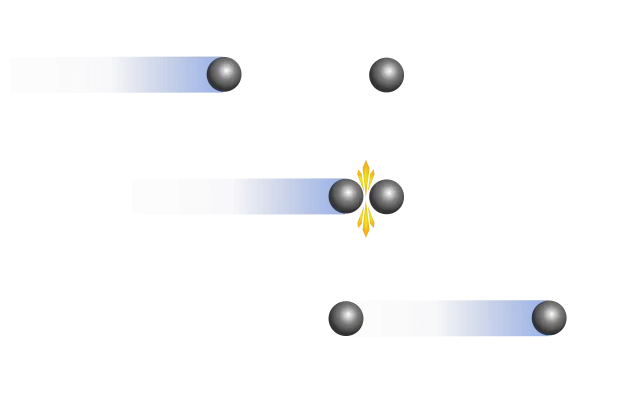[Image 1]

## Introduction

Hey it's a me again @drifter1! Today we continue with Physics and more specifically the branch "Classical Mechanics" to get into some Examples of Collisions. So, without further ado, let's get straight into it!

## Elastic Collisions

As we said in the previous article, Elastic Collisions are those where both the Momentum and Kinetic Energy are being conserved. Analysing such a collision mathematically we found some very useful equations that can be used when we are trying to calculate the velocity of each object that took part at the collision after the collision. Based on all that, let's get into some examples now...

### 1. An object collides with an object at rest

Based on an example from varsitytutors

Consider the following situtation:Knowing the mass of both objects, the initial and final velocity of the first one, and that the second (heavier) object is at rest first, let's calculate the final velocity of the second object.

First of all, it's not a special case like the ones that we described last time, cause here the two objects don't have equal masses and the difference in mass is also not quite large (to talk about a light and heavy object). So, what do we do? With the information that is given to us, we just have to apply the conservation of momentum for the system before and after the collision. That way we have:Knowing that the velocity of object 2 is zero at first, we can eliminate this component from the equation, and afterwards find the final velocity of the second object, by solving the equation for v2' and putting the given values for each quantity:We could make even more examples with different given quantities...

### 2. Two objects of equal mass move in the same direction

Based on an example from spiff.rit.edu

Let's suppose that we have two balls of mass m = 0.2kg that move in the same direction. The ball in front is moving slower than the ball behind it and so the second ball catches up and hits it. If we know that the speed of each of them is v1 = 1 m/s and v2 = 3 m/s, what happens after they hit?

You might remember that this is a special case. From that special case we can already say that the two balls will "exchange" speed. So, both of them will still go in the same direction, but now the ball in front will be faster than the ball behind it, moving at v1' = v2 = 3 m/s, while the ball behind will go slower at an velocity of v2' = v1 = 1 m/s. Let's prove these values using the two equations that came out applying both the conservation of momentum and kinetic energy:Having equal masses one part of each equation gets eliminated:So, in the end we end up with:You can see that they truly exchange velocities!

### 3. Momentum Conservation of 1.

To understand elastic collisions even better let's get into the first example more in-depth. To do so, let's calculate the momentum values before and after the collision, for each object and the total system, to see how the energy and momentum moves from one object to the other.

The initial momentum of the system is equal to the initial momentum of the moving object, whilst the initial momentum of the object at rest is zero. Mathematically we have the following momentum values:After the collision some momentum of the moving object is given to the stationary one, keeping the total momentum of the system the same. And so we have:## Inelastic Collision

After those simpler elastic collisions let's now also get into an inelastic collision example!

### A truck "locks" onto a stationary car

Example from sciencenotes

A 3000 kg truck travelling at 50 km/h strikes a stationary 1000 kg car, locking the two vehicles together.
Calculate:
1. The final velocity V of the two vehicles
2. How much kinetic energy is lost in the collision?
1.
Knowing that the two vehicles form a single "object" after the collision (aggregation), we can say that this collision is inelastic. So, we can only apply the conservation of momentum. By applying it we get:2.
The initial kinetic energy is: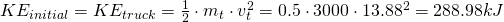The final kinetic energy, after the collision, is: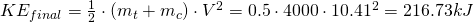Therefore the loss in kinetic energy is: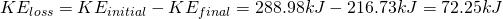As an percentage this can be represented as:
So, roughly 3/4 of the initial kinetic energy are being conserved, or we can also say that 1/4 of the energy is being lost.

## RESOURCES:

### References

1. https://www.varsitytutors.com/high_school_physics-help/understanding-elastic-and-inelastic-collisions
2. http://spiff.rit.edu/classes/phys311.old/lectures/elas/elas.html
3. https://sciencenotes.org/inelastic-collision-example-problem-physics-homework-help/

### Images

1.## Final words | Next up

This is actually it for today's post! Next time we will get into Impulse..

See ya!Keep on drifting!

H2
H3
H4
3 columns
2 columns
1 column